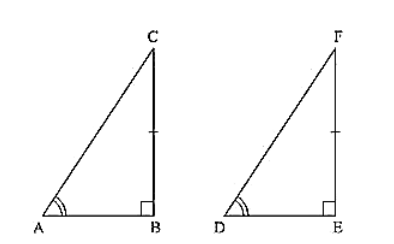# In two right triangles one side an acute angle of one are equal to the corresponding side and angle of the other. Prove that the triangles are congruent.

Question:

In two right triangles one side an acute angle of one are equal to the corresponding side and angle of the other. Prove that the triangles are congruent.

Solution:

Given that, in two right triangles one side and acute angle of one are equal to the corresponding side and angles of the other.We have to prove that the triangles are congruent.

Let us consider two right triangles such that

∠B = ∠C = 90° .... (i)

AB = DE .... (ii)

∠C = ∠F       From(iii)

Now observe the two triangles ABC and DEF

∠C = ∠F [From (iii)]

∠B = ∠E [From (i)]

and AB = DE   [From (ii)]

So, by AAS congruence criterion, we have ΔABC ≅ ΔDEF

Therefore, the two triangles are congruent

Hence proved